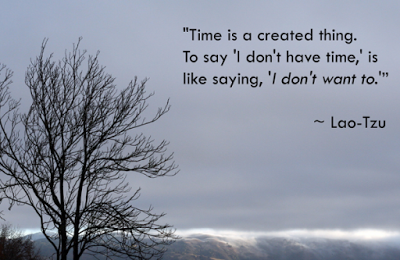## Tuesday, 1 September 2015If you are sitting on a couch at home, then you are not moving i.e. having zero velocity relative to your home and Earth, but you are revolving around Sun relative to Sun. If you are going on a bike, then you have zero velocity relative to your bike because you are going on the bike, but you possess some velocity relative to Earth. The geosynchronous satellite which is always at the same location of Earth's atmosphere is relatively having zero velocity relative to Earth, but it rotates along with Earth and revolves around Sun relative to Sun.

See, there is a difference in how Speed of an object is relative. We know Speed = Distance Travelled ÷ Time. See Speed is relative. Distance traveled is relatively constant. Looking at the equation, Speed [relative] = Relatively traveled Distance ÷ Time, we derive Time = Relatively traveled Distance ÷ Speed [relative]. From the last equation, we see Time is the outcome of Relatively traveled Distance and Speed, which is relative. That is why I say Time is not a constant, but relatively variable.